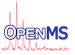OpenMS
RANSACParam Struct Reference

A simple struct to carry all the parameters required for a RANSAC run. More...

#include <OpenMS/MATH/MISC/RANSAC.h>

Collaboration diagram for RANSACParam:
[legend]

## Public Member Functions

RANSACParam ()
Default constructor. More...

RANSACParam (size_t p_n, size_t p_k, double p_t, size_t p_d, bool p_relative_d=false)
Full constructor. More...

std::string toString () const

## Public Attributes

size_t n
data points: The minimum number of data points required to fit the model More...

size_t k
iterations: The maximum number of iterations allowed in the algorithm More...

double t
Threshold value: for determining when a data point fits a model. Corresponds to the maximal squared deviation in units of the _second_ dimension (dim2). More...

size_t d
The number of close data values (according to 't') required to assert that a model fits well to data. More...

bool relative_d
Should 'd' be interpreted as percentages (0-100) of data input size. More...

## Detailed Description

A simple struct to carry all the parameters required for a RANSAC run.

## ◆ RANSACParam() [1/2]

 RANSACParam ( )
inline

Default constructor.

## ◆ RANSACParam() [2/2]

 RANSACParam ( size_t p_n, size_t p_k, double p_t, size_t p_d, bool p_relative_d = false )
inline

Full constructor.

References RANSACParam::d, and RANSACParam::relative_d.

## ◆ toString()

 std::string toString ( ) const
inline

## ◆ d

 size_t d

The number of close data values (according to 't') required to assert that a model fits well to data.

Referenced by RANSAC< TModelType >::ransac(), RANSACParam::RANSACParam(), and RANSACParam::toString().

## ◆ k

 size_t k

iterations: The maximum number of iterations allowed in the algorithm

Referenced by RANSAC< TModelType >::ransac(), and RANSACParam::toString().

## ◆ n

 size_t n

data points: The minimum number of data points required to fit the model

Referenced by RANSAC< TModelType >::ransac(), and RANSACParam::toString().

## ◆ relative_d

 bool relative_d

Should 'd' be interpreted as percentages (0-100) of data input size.

Referenced by RANSAC< TModelType >::ransac(), and RANSACParam::RANSACParam().

## ◆ t

 double t

Threshold value: for determining when a data point fits a model. Corresponds to the maximal squared deviation in units of the _second_ dimension (dim2).

Referenced by RANSAC< TModelType >::ransac(), and RANSACParam::toString().